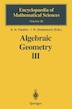Algebraic Geometry III: Complex Algebraic Varieties Algebraic Curves and Their Jacobians

byV.s. Kulikov, I. Rivin, V.v. Shokurov

Pricing and Purchase Info

\$199.12 online
\$212.95 list price save 6%
Earn 996 plum® points

Prices and offers may vary in store

Quantity:

In stock online

Ships free on orders over \$25

Not available in stores

Starting with the end of the seventeenth century, one of the most interesting directions in mathematics (attracting the attention as J. Bernoulli, Euler, Jacobi, Legendre, Abel, among others) has been the study of integrals of the form r dz l Aw(T) = -, TO W where w is an algebraic function of z. Such integrals are now called abelian. Let us examine the simplest instance of an abelian integral, one where w is defined by the polynomial equation (1) where the polynomial on the right hand side has no multiple roots. In this case the function Aw is called an elliptic integral. The value of Aw is determined up to mv + nv , where v and v are complex numbers, and m and n are 1 2 1 2 integers. The set of linear combinations mv+ nv forms a lattice H C C, and 1 2 so to each elliptic integral Aw we can associate the torus C/ H. 2 On the other hand, equation (1) defines a curve in the affine plane C = 2 2 {(z,w)}. Let us complete C2 to the projective plane lP' = lP' (C) by the addition of the "line at infinity", and let us also complete the curve defined 2 by equation (1). The result will be a nonsingular closed curve E C lP' (which can also be viewed as a Riemann surface). Such a curve is called an elliptic curve.
Title:Algebraic Geometry III: Complex Algebraic Varieties Algebraic Curves and Their JacobiansFormat:PaperbackDimensions:270 pagesPublished:December 1, 2010Publisher:Springer-Verlag/Sci-Tech/TradeLanguage:English

The following ISBNs are associated with this title:

ISBN - 10:3642081185

ISBN - 13:9783642081187